• +91 9971497814
• info@interviewmaterial.com

# Surface Areas and Volumes Ex-13.2 Interview Questions Answers

### Related Subjects

Question 1 :

A solid is in theshape of a cone standing on a hemisphere with both their radii being equal to 1cm and the height of the cone is equal to its radius. Find the volume of thesolid in terms of π.

Answer 1 :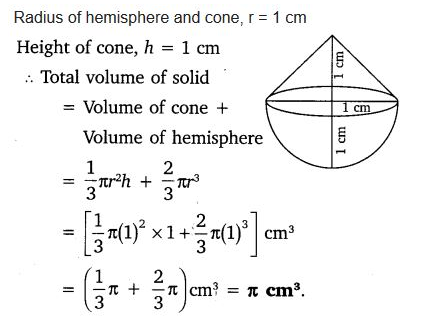Question 2 :

Rachel, anengineering student, was asked to make a model shaped like a cylinder with twocones attached at its two ends by using a thin aluminum sheet.

Answer 2 : The diameter of the model is 3 cm and its length is 12 cm. If each cone has a height of 2 cm, find the volume of air contained in the model that Rachel made. (Assume the outer and inner dimensions of the model to be nearly the same.)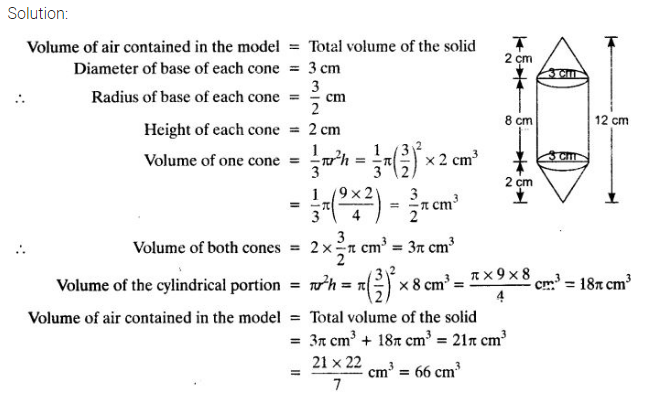Question 3 : A Gulab jamun contains sugar syrup up to about30% of its volume. Find approximately how much syrup would be found in 45 Gulabjamuns,

Answer 3 :  each shaped like a cylinder with two hemispherical ends with length 5 cm and diameter 2.8 cm (see figure).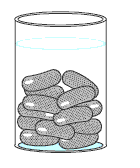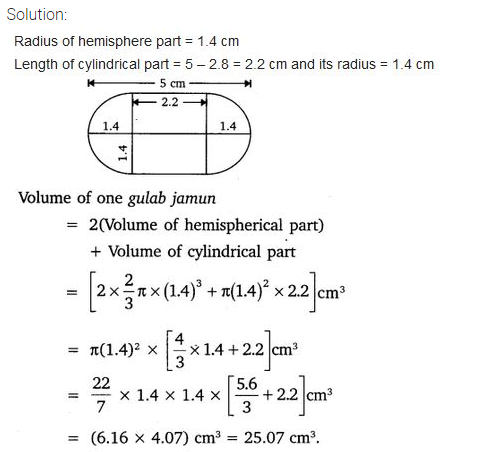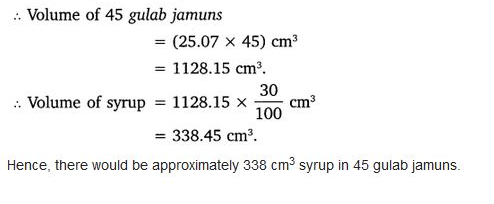Question 4 :

A pen stand made ofwood is in the shape of a cuboid with four conical depressions to hold pens.The dimensions of the cuboid are 15 cm by 10 cm by 3.5 cm.

Answer 4 : The radius of each of the depressions is 0.5 cm and the depth is 1.4 cm. Find the volume of wood in the entire stand (see Fig.).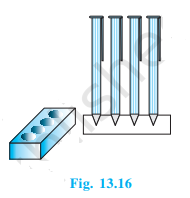Solution:

Volume of cuboid = length x width x height

We know the cuboid’s dimensions as 15 cmx10cmx3.5 cm

So, the volume of the cuboid = 15x10x3.5 = 525cm3

Here, depressions are like cones and we know,

Volume of cone = (⅓)πr2h

Given, radius (r) = 0.5 cm and depth (h) = 1.4cm

Volume of 4 cones = 4x(⅓)πr2h

= 1.46 cm2

Now, volume of wood = Volume of cuboid – 4 xvolume of cone

= 525-1.46 = 523.54 cm2

Question 5 :

A vessel is in theform of an inverted cone. Its height is 8 cm and the radius of its top, whichis open, is 5 cm. It is filled with water up to the brim. When lead shots,

Answer 5 :  each of which is a sphere of radius 0.5 cm are dropped into the vessel, one-fourth of the water flows out. Find the number of lead shots dropped in the vessel.

Solution:

For the cone,

Height = 8 cm

Also,

Radius of sphere = 0.5 cm

The diagram will be like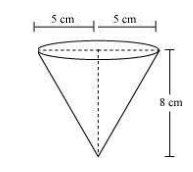It is known that,

Volume of cone = volume of water in the cone

= ⅓πr2h = (200/3)π cm3

Now,

Total volume of water overflown= (¼)×(200/3) π=(50/3)π

= (4/3)πr3

= (1/6) π

Now,

The number of lead shots = Total Volume ofWater over flown/ Volume of Lead shot

= (50/3)π/(

= (50/3)×6 = 100

Question 6 : A solid iron pole consists of a cylinder ofheight 220 cm and base diameter 24 cm, which is surmounted by another cylinderof height 60 cm and radius 8 cm. Find the mass of the pole, given that 1 cm3 ofiron has approximately 8 g mass.

Given, the height of the big cylinder (H) =220 cm

Radius of the base (R) = 24/12 = 12 cm

So, the volume of the big cylinder = πR2H

= π(12)× 220 cm3

= 99565.8 cm3

Now, the height of smaller cylinder (h) = 60cm

Radius of the base (r) = 8 cm

So, the volume of the smaller cylinder = πr2h

= π(8)2×60 cm3

= 12068.5 cm3

Volume of iron = Volume of the big cylinder+ Volume of thesmall cylinder

= 99565.8 + 12068.5

=111634.5 cm3

We know,

Mass = Density x volume

So, mass of the pole = 8×111634.5

= 893 Kg (approx.)

Question 7 : A solid consisting of a right circular cone ofheight 120 cm and radius 60 cm standing on a hemisphere of radius 60 cm isplaced upright in a right circular cylinder full of water such that it touchesthe bottom. Find the volume of water left in the cylinder, if the radius of thecylinder is 60 cm and its height is 180 cm.